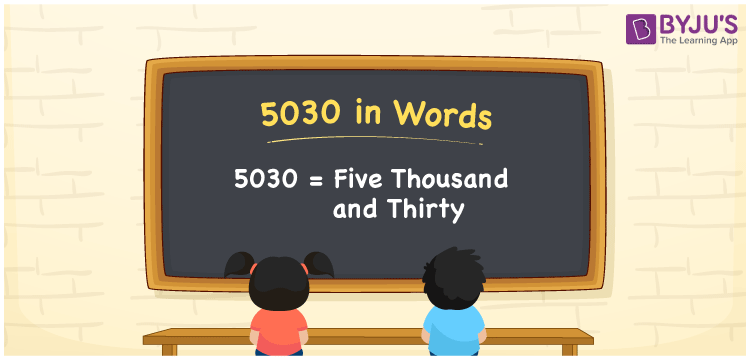# 5030 in words

5030 in words is written as Five Thousand and Thirty. 5030 represents the count or value. The article on Counting Numbers can give you an idea about count or counting. The number 5030 is used in expressions that relate to money, distance, length, year and others. Let us consider an example for 5030. ”Five Thousand and Thirty meters is slightly more than Five kilometers”.

 5030 in words Five Thousand and Thirty Five Thousand and Thirty in Numbers 5030

## 5030 in English Words## How to Write 5030 in Words?

We can convert 5030 to words using a place value chart. The number 5030 has 4 digits, so let’s make a chart that shows the place value up to 4 digits.

 Thousands Hundreds Tens Ones 5 0 3 0

Thus, we can write the expanded form as:

5 × Thousand + 0 × Hundred + 3 × Ten + 0 × One

= 5 × 1000 + 0 × 100 + 3 × 10 + 0 × 1

= 5030

= Five Thousand and Thirty.

5030 is the natural number that is succeeded by 5029 and preceded by 5031.

5030 in words – Five Thousand and Thirty.

Is 5030 an odd number? – No.

Is 5030 an even number? – Yes.

Is 5030 a perfect square number? – No.

Is 5030 a perfect cube number? – No.

Is 5030 a prime number? – No.

Is 5030 a composite number? – Yes.

## Solved Example

1. Write the number 5030 in expanded form

Solution: 5 × 1000 + 0 × 100 + 3 × 10 + 0 × 1

We can write 5030 = 5000 + 000 + 30 + 0

= 5 × 1000 + 0 × 100 + 3 × 10 + 0 × 1

## Frequently Asked Questions on 5030 in words

Q1

### How to write 5030 in words?

5030 in words is written as Five Thousand and Thirty.
Q2

### Is 5030 a perfect square number?

No. 5030 is not a perfect square number.
Q3

### Is 5030 a prime number?

No. 5030 is not a prime number.